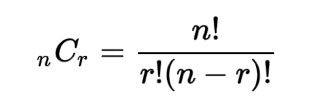Request a Tool

Combination Calculator

Combination Calculator use to calculate combination from n and r values.

Combination
0

Formula• C = Combination
• n = Total number of objects in the set
• r = Number of choosing objects from the set

Defination / Uses

A combination in mathematics is a selection of items from a collection where the order of selection is irrelevant (unlike permutations).

The act of selecting items from a collection in such a way that the order in which they are chosen is irrelevant. Combinations of those numbers refer to the number of different groups that can be formed by picking some or all of the components. Combinatorics is an area of mathematics that includes both permutations and combinations. Combinations and permutations are not the same as rounding and midpoint. Our Rounding Calculator and Midpoint Calculator might help you understand these topics.